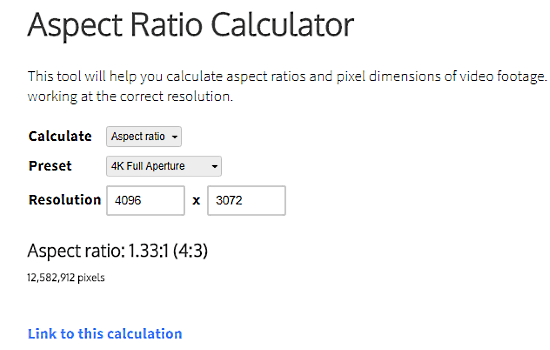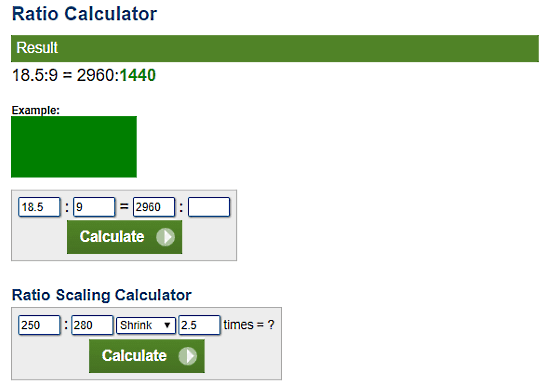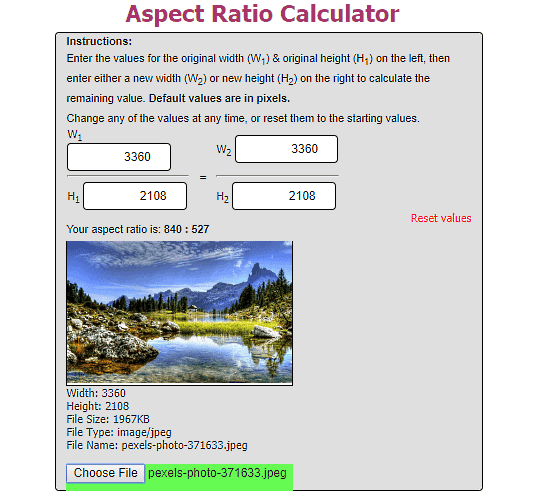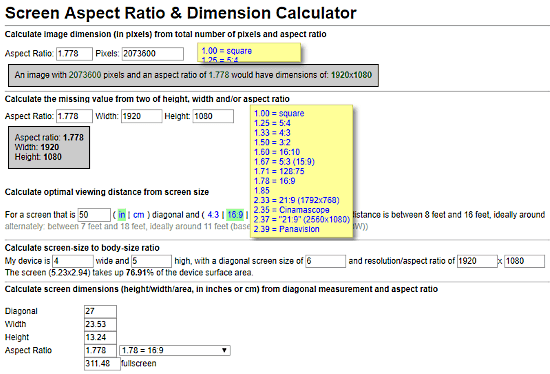Editor Ratings:
User Ratings:
[Total: 0 Average: 0]

This article talks about 5 free Screen Aspect Ratio Calculator websites where you can calculate the aspect ratio of a video or screen. The aspect ratio of a video/screen is the ratio of height and width of its resolution/dimension.

These aspect ratio calculators take resolution as input and calculate the aspect ratio for that resolution. Most of these calculators can also calculate resolution from aspect ratio as well.

For further queries and questions about Aspect Ratio, you can read our article on What is Aspect Ratio? [ILFS Explains].

## Digital Rebellion.comDigital Rebellion.com offers a free tool that helps you calculate the aspect ratio of a video or screen. You can do so either by selecting the preset values or by entering the resolution manually. For example, in the screenshot above, I manually enter the 4K resolution (which is also present in the preset), and it calculated the aspect ratio for that resolution, which is 1.33:1 (4:3 as by the standards). Along with aspect ratio, it also shows you the number of pixels in that resolution.

Try this Aspect Ratio Calculator here.

## Andrew.Hedges.nameAndrew.Hedges.name‘s Aspect Ratio Calculator calculates aspect ratio by taking width and height as input. Simply enter the width and height of your video file or screen and it will calculate the aspect ratio for that. You can see the exact aspect ratio or can round off the results to the nearest whole number. This calculator shows you a sample image in that aspect ratio.

Here, you can also calculate another resolution for the same aspect. For that, enter either height or width for the new resolution and it will calculate the other one for you. This way, you can make any desired resolution in the same aspect ratio.

Know your screen aspect ratio with Andrew.Hedges.name here.

## Calculator.netCalculator.net offers an online Ratio Calculator that calculates aspect ratio from resolution. You can calculate any desired aspect ratio by giving it a value of that aspect ratio along with the resolution. It can do the same for resolution as well. For example, here I gave it the aspect ratio of Samsung Galaxy S8 (18.5:9) along with resolution height (2960) and it calculated the resolution width (which is 1440) for that.

It also has a Ratio Scale Calculator where you can shrink/enlarge a resolution or aspect ratio by any value. And, it also shows you a relative visual comparison of before and after scaling.

Calculate your screen aspect ratio here.

## BellevueFineArt.comBellevueFineArt.com

This Aspect Ratio Calculator offers the same functionality as Andrew.Hedges.name’s Aspect Ratio Calculator. Here, you can calculate aspect ratio from resolution. You can also generate a new resolution with that same aspect ratio. For that, enter any desired height or width for the new resolution and it will calculate the other missing dimension for that new resolution.

Apart from that, you can upload photos from computer to calculate their resolution and aspect ratio here.

Try this Aspect Ratio Calculator here.

## SiliSoftware.comSiliSoftware.com offers a multi-purpose Dimension & Aspect Ratio Calculator tool. With this tool, you can calculate:

• Resolution along with the total number of pixels from aspect ratio.
• Missing value of height, width or aspect ratio when any two of them are known.
• Optimal viewing distance from screen size for any screen size.
• Screen-size to body-size ratio (ratio of the area of a screen and the area of the whole front side of the device including bezels).
• Height, width and area of a screen from its diagonal value and aspect ratio.

Calculate your screen ratio & dimensions with SiliSoftware.com‘s calculator here.

### Final Verdict:

All these Aspect Ratio Calculator websites are simple and easy to use. You can use any of these calculators to know the aspect ratio of a video file or a screen.

 Editor Ratings: User Ratings:[Total: 0 Average: 0] Free/Paid: Free Tags: calculator